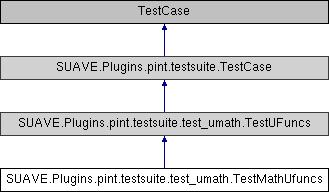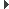SUAVE  2.5.2 An Aerospace Vehicle Environment for Designing Future AircraftSUAVE.Plugins.pint.testsuite.test_umath.TestMathUfuncs Class Reference
Inheritance diagram for SUAVE.Plugins.pint.testsuite.test_umath.TestMathUfuncs:## Public Member Functions

def test_subtract (self)

def test_multiply (self)

def test_divide (self)

def test_true_divide (self)

def test_floor_divide (self)

def test_negative (self)

def test_remainder (self)

def test_mod (self)

def test_fmod (self)

def test_absolute (self)

def test_rint (self)

def test_conj (self)

def test_exp (self)

def test_exp2 (self)

def test_log (self)

def test_log2 (self)

def test_log10 (self)

def test_expm1 (self)

def test_sqrt (self)

def test_square (self)

def test_reciprocal (self)Public Member Functions inherited from SUAVE.Plugins.pint.testsuite.test_umath.TestUFuncs
def qless (self)

def qs (self)

def q1 (self)

def q2 (self)

def qm (self)

def qi (self)

def assertEqual (self, first, second, msg=None)

def assertRaisesMsg (self, msg, ExcType, func, *args, **kwargs)Public Member Functions inherited from SUAVE.Plugins.pint.testsuite.TestCase
def setUpClass (cls)

def assertSequenceEqual (self, seq1, seq2, msg=None, seq_type=None)

def assertAlmostEqual (self, first, second, places=None, msg=None, delta=None)

def assertAlmostEqualRelError (self, first, second, rel, msg=None)Public Attributes inherited from SUAVE.Plugins.pint.testsuite.TestCase
ureg

Q_Static Public Attributes inherited from SUAVE.Plugins.pint.testsuite.test_umath.TestUFuncs
bool FORCE_NDARRAY = True

## Detailed Description

```Universal functions (ufunc) > Math operations

http://docs.scipy.org/doc/numpy/reference/ufuncs.html#math-operations

subtract(x1, x2[, out])     Subtract arguments, element-wise.
multiply(x1, x2[, out])     Multiply arguments element-wise.
divide(x1, x2[, out])   Divide arguments element-wise.
logaddexp(x1, x2[, out])    Logarithm of the sum of exponentiations of the inputs.
logaddexp2(x1, x2[, out])   Logarithm of the sum of exponentiations of the inputs in base-2.
true_divide(x1, x2[, out])  Returns a true division of the inputs, element-wise.
floor_divide(x1, x2[, out])     Return the largest integer smaller or equal to the division of the inputs.
negative(x[, out])  Returns an array with the negative of each element of the original array.
power(x1, x2[, out])    First array elements raised to powers from second array, element-wise. NOT IMPLEMENTED
remainder(x1, x2[, out])    Return element-wise remainder of division.
mod(x1, x2[, out])  Return element-wise remainder of division.
fmod(x1, x2[, out])     Return the element-wise remainder of division.
absolute(x[, out])  Calculate the absolute value element-wise.
rint(x[, out])  Round elements of the array to the nearest integer.
sign(x[, out])  Returns an element-wise indication of the sign of a number.
conj(x[, out])  Return the complex conjugate, element-wise.
exp(x[, out])   Calculate the exponential of all elements in the input array.
exp2(x[, out])  Calculate 2**p for all p in the input array.
log(x[, out])   Natural logarithm, element-wise.
log2(x[, out])  Base-2 logarithm of x.
log10(x[, out])     Return the base 10 logarithm of the input array, element-wise.
expm1(x[, out])     Calculate exp(x) - 1 for all elements in the array.
log1p(x[, out])     Return the natural logarithm of one plus the input array, element-wise.
sqrt(x[, out])  Return the positive square-root of an array, element-wise.
square(x[, out])    Return the element-wise square of the input.
reciprocal(x[, out])    Return the reciprocal of the argument, element-wise.
ones_like(x[, out])     Returns an array of ones with the same shape and type as a given array.
```

The documentation for this class was generated from the following file:
• /Users/emiliobotero/Dropbox/SUAVE/SUAVE/trunk/SUAVE/Plugins/pint/testsuite/test_umath.py